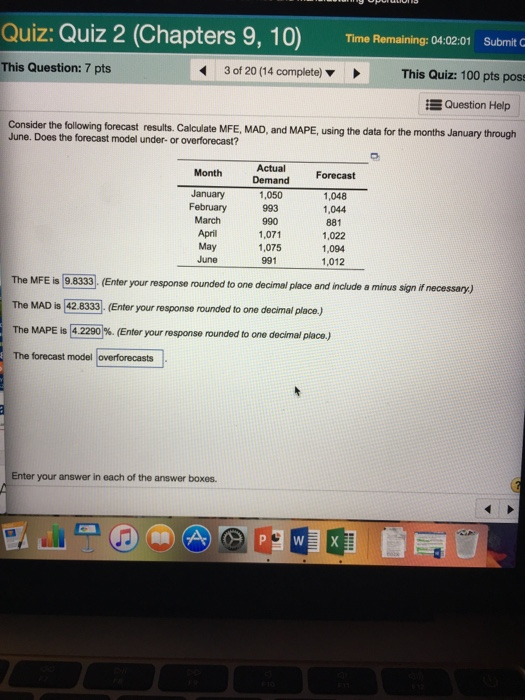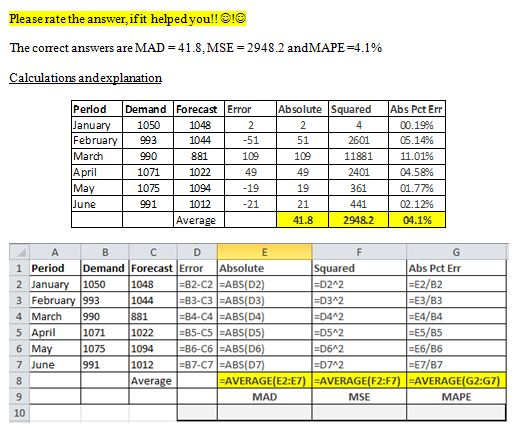# Question & Answer: Consider the following forecast results. Calculate MFE, MAD, and MAPE, using…..Consider the following forecast results. Calculate MFE, MAD, and MAPE, using the data for the months January through June. Does the forecast model under- or overforecast? The MFE is 9.8333. (Enter your response rounded to one decimal place and include a minus sign necessary.) The MAD is 42.8333. (Enter your response rounded to one decimal place.) The MAPE is 4.2290%. (Enter your response rounded to one decimal place.) The forecast model overforecasts.